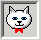## Monday, August 17, 2009

### 2+2 = 4199232

2+2 = 4199232

If only C++ was not so notorious !

Trying out this simple program in Quincy gives a weird output !

What is happening is that, The printf statement: printf("The answer is %d\n"); tells the program to print an integer, but fails to supply one. The printf function doesn't know this, so it will take the next number off the stack (some random number) and print it.

The correct code should be: printf("The answer is %d\n", answer);

Running the program in Unix (Ubuntu) gave another result ! 2+2 = 134518880

Fig 3. 2+2 = 134518880REFERENCES

(1) Oualinne, Steve "How not to Program in C++", No Starch Press 2003
(2) Quincy